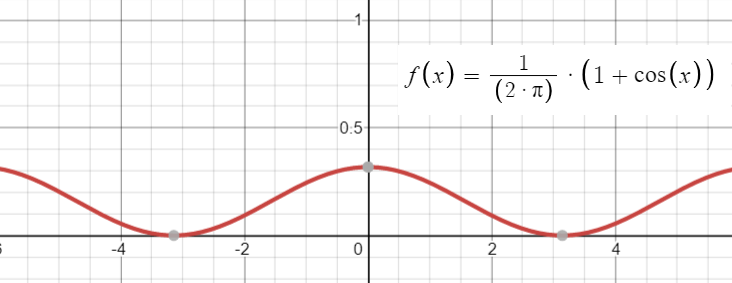# Cosine Distribution

Share on

The cosine distribution is an approximation to the normal distribution. Using a cosine distribution instead of a normal can simplify algebraic manipulations. For example, expressions with products or powers of the normal probability density function and its integral are difficult to evaluate but the cosine distribution is much easier to work with in this regard. Numerical evaluations stay the same and can be interpreted with trigonometric tables .

The cosine is platykurtic compared to a normal distribution, so the fit isn’t exact. However, it does give reasonable approximations. For example, a cosine function shifted up one period with the area under the curve normalized to 1 is can approximate a normal probability distribution in the range ± 2.5 standard deviations with an accuracy of about ±0.02 .

Cosine distributions are used for a wide range of applications including fields as diverse as psychological investigations , semiconductor manufacturing, atomic sputtering, and antenna design.

## Cosine Distribution PDF

The probability density function (PDF) for the cosine distribution is :
Cosine PDF(x) = 1/(2 * π) * (1 + cos(x)).## References

Cosine graph created with Desmos.
 Raab, D. & Green, E. (1961). A cosine approximation to the normal distribution. Psychometrika, Vol. 26, No. 4. Retrieved December 19, 2021 from: https://link.springer.com/content/pdf/10.1007/BF02289774.pdf
 Warsza, Z. & Korczynski, J. (2010). Shifted up cosine function as unconventional model of probability distribution. Journal of Automation, Mobile Robotics & Intelligent Systems. Volume 4, No.1.
 Scipy.stats.cosine. Retrieved December 19, 2021 from: https://het.as.utexas.edu/HET/Software/Scipy/generated/scipy.stats.cosine.html

CITE THIS AS:
Stephanie Glen. "Cosine Distribution" From StatisticsHowTo.com: Elementary Statistics for the rest of us! https://www.statisticshowto.com/cosine-distribution/
---------------------------------------------------------------------------Need help with a homework or test question? With Chegg Study, you can get step-by-step solutions to your questions from an expert in the field. Your first 30 minutes with a Chegg tutor is free!